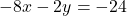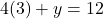Question

Solve by substitution. Show each step of your work.

4x + y = 12

6x + 2y = 18

in progress 0
2 months 2021-08-01T04:07:12+00:00 2 Answers 2 views 0

x = 3, y = 0

Step-by-step explanation:

First we find a variable to cancel out. Y will be easiest.

Multiply that equation by -2 to createNow we can cancel y out and solve for x.

add the variables together to make 10x = 30

x = 3

Now that we have x, we can plug it in for either equation to find y.y = 0

2. 5x + y = 15

y = -5x + 15

Substituting y= -5x+15 from first equation into second equation:

3x + 2y = 16

3x + 2·(-5x + 15) = 16

3x – 10x + 30 = 16

-7x + 30 = 16

7x = 30 – 16 = 14

x = 2

Substituting x=2 into the first equation:

5x + y = 15

5(2) + y = 15

10 + y = 15

y = 15 – 10

y = 5